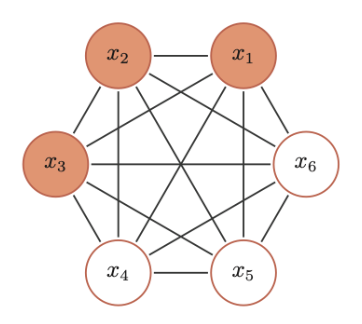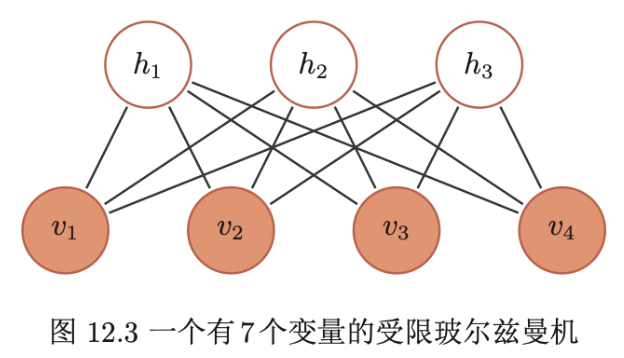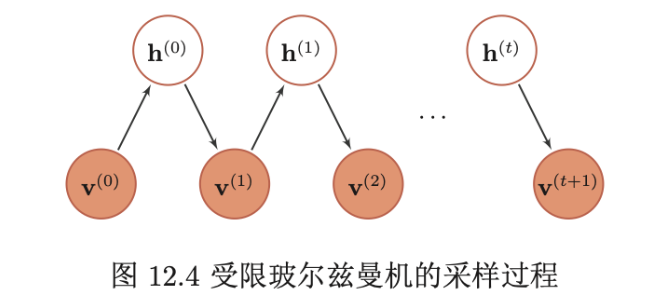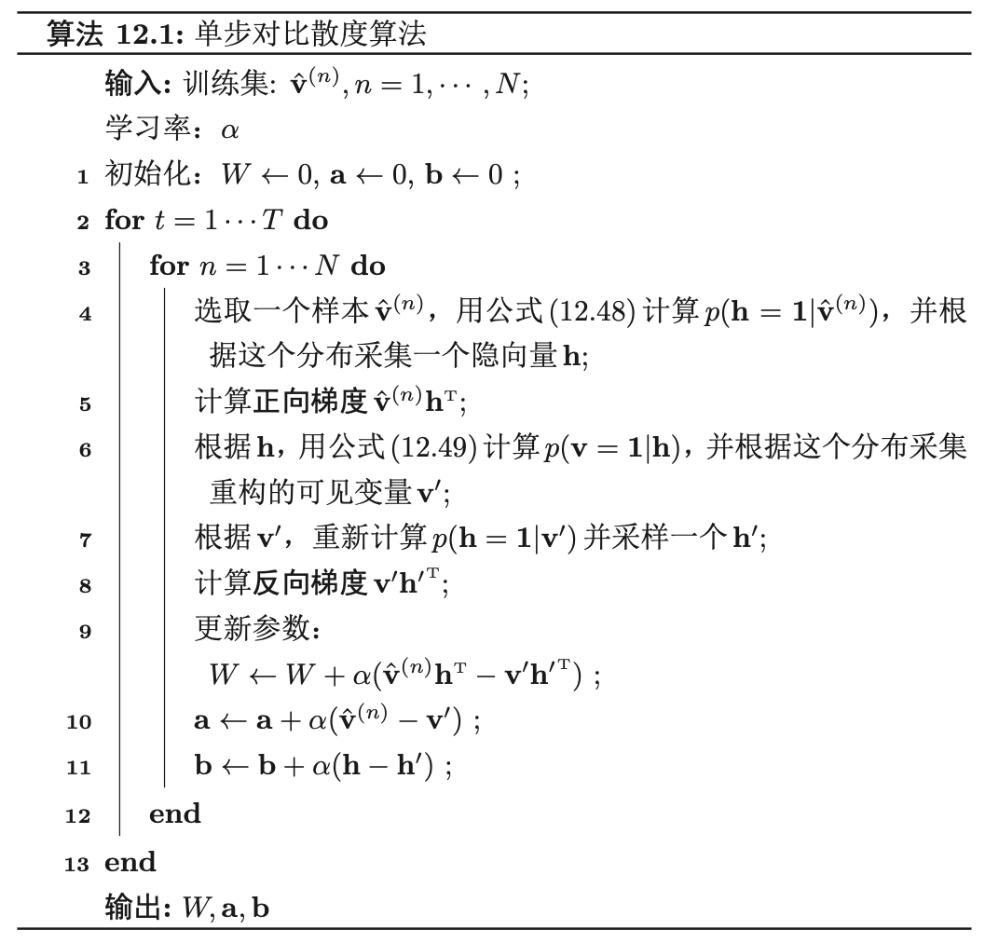Github：https://github.com/thinkgamer

• 背景
• 玻尔兹曼机
• 受限玻尔兹曼机

### 玻尔兹曼机

#### 介绍BM的三个性质：

• 二值输出：每个随机变量可以用一个二值的随机变量表示
• 全连接：所有节点之间是全连接的
• 权重对称：每两个变量之间的相互影响是对称的

BM中的每个变量$X$的联合概率由玻尔兹曼分布得到，即：

1. 全条件概率

2. 吉布斯采样

3. 能量最小化与模拟退火

### 受限玻尔兹曼机

#### 介绍• 可观测的随机向量$v=[v_1, …, v_{m_1}]^T$
• 隐藏的随机向量 $h=[h_1, … , h_{m_2}]^T$
• 权重矩阵$W \in R^{m_1 * m_2}$，其中每个元素$w_{ij}$为可观测变量$v_i$和隐变量$h_j$之间边的权重
• 偏置$a \in R^{m_1}$和$b \in R^{m_2}$，其中$a_i$为每个可观测变量$v_i$得偏置，$b_j$为每个隐变量$h_j$得偏置

#### 生成模型

1. 全条件概率

2. RBM中得吉布斯采样

• （给定）或随机初始化一个可观测的向量$v_0$，计算隐变量得概率，并从中采样一个隐向量$h_0$
• 基于$h_0$，计算可观测变量得概率，并从中采样一个个可观测的向量$v_1$
• 重复$t$次后，获得$(v_t, h_t)$
• 当$t \rightarrow \infty$时，$(v_t,h_t)$的采样服从$p(v,h)$分布3. 对比散度学习算法4. 受限玻尔兹曼机得类型

• “伯努利-伯努利”受限玻尔兹曼机：即上面介绍得可观测变量喝隐变量都为二值类型得受限玻尔兹曼机
• “高斯-伯努利”受限玻尔兹曼机：假设可观测变量为高斯分布，隐变量为伯努利分布，其能量函数定义为：其中每个可观测变量$v_i$服从$(\mu_i, \sigma_i)$的高斯分布。
• “伯努利-高斯”受限玻尔兹曼机：假设可观测变量为伯努利分布，隐变量为高斯分布，其能量函数定义为：其中每个隐变量$h_j$服从$(\mu_j, \sigma_j)$的高斯分布

【技术服务】，详情点击查看： https://mp.weixin.qq.com/s/PtX9ukKRBmazAWARprGIAg